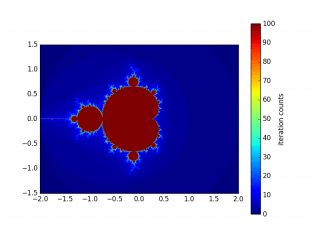Please enable Javascript to view the contents

·  ☕ 4 分钟

## 1. Koch曲线

1. 指定一条线段的长度$$l$$（可以理解为第0次迭代）
2. 将这条线段三等分，并以中间的线段为底边构造一个等边三角形，然后去掉底边
3. 对2中生成的曲线的每一条边重复2的操作（每操作一次称为一次迭代）

### 1.2 绘制方法：

1. 如果N=0，直接画出L长的直线即可
2. 如果N=1（第一次迭代），画出长度为L/3的线段；画笔向左转60度再画长度为L/3长的线段；画笔向右转120度画长度为L/3长的线段；画笔再向左转60度画出长度为L/3的线段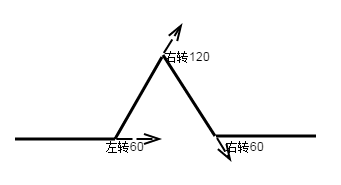1. 如果n>1，第n次迭代相当于：n-1次迭代；画笔左转60度；n-1次迭代；画笔右转120度；n-1次迭代；画笔左转60度；n-1次迭代。

### 1.3 Python代码实现

  1 2 3 4 5 6 7 8 9 10 11 12 13 14 15 16 17 18 19 20 21 22 23  # -*- coding: utf-8 -*- import turtle Division = 3.0 DirectionAangle = [('left',60),('right',120),('left',60)] def call(name): if name == 'left': return turtle.left else: return turtle.right def koch(n, length): if n==0: turtle.forward(length) else: for DA in DirectionAangle: koch(n-1,length/Division) call(DA)(DA) koch(n-1,length/Division) koch(n=2, length=100) turtle.done() 

### 1.4 绘制的图形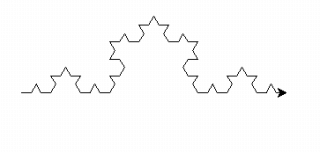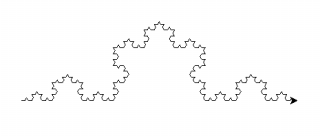## 2. Julia集

### 2.1 绘制方法

1. 设定初值 p,q, 最大的迭代次数 N, 图形的大小 a,b, 及使用的颜色数 K.这里需要注意的是c的模总是小于2。可以证明当c的模大于2时，进行迭代必将发散到无穷。
2. 设定区域的界值 $$M\ge max(2,\sqrt{p^2+q^2})$$
3. 将区域$$R=[-M,M]\times[-M,M]$$分成$$a\times b$$的网格，分别以每个网格点为初值($$x_0,y_0$$)。利用上面替换之后的公式做迭代。如果对$$n \le N$$所有的都有$${x_n}^2+{y_n}^2\le M^2$$，则将象素$$(i, j)$$置为这一种颜色。如果从某一步 n 开始$${x_n}^2+{y_n}^2\ge M^2$$，则将象素 $$(i, j)$$置为不同颜色。

### 2.2 Python代码实现

  1 2 3 4 5 6 7 8 9 10 11 12 13 14 15 16 17 18 19 20 21 22 23 24 25 26 27 28 29 30  # -*- coding: utf-8 -*- import matplotlib.pyplot as plot import numpy as np p=0.45 #初始值c的实部 q=-0.1428 #初始值c的虚部 N=800 #最大迭代次数 M=100 #迭代区域的界值 a=3.0 #绘制图的横轴大小 b=3.0 #绘制图的纵轴大小 step=0.005 #绘制点的步长 def iterate(z,N,M): z=z*z+c for i in xrange(N): if abs(z)>M: return i z=z*z+c return N c=p+q*1j i=np.arange(-a/2.0,a/2.0,step) j=np.arange(b/2.0,-b/2.0,-step) I,J=np.meshgrid(i, j) ufunc=np.frompyfunc(iterate,3,1) Z=ufunc(I+1j*J,N,M).astype(np.float) plot.imshow(Z,extent=(-a/2.0,a/2.0,-b/2,b/2.0)) cb = plot.colorbar(orientation='vertical',shrink=1) cb.set_label('iteration counts') plot.show() 

### 2.3 绘制的图形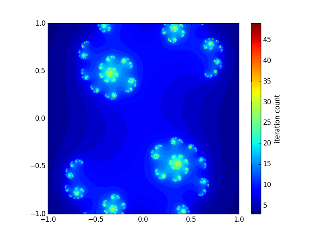c = -0.70176+-0.3842j
c = -0.835+-0.2321j
c = -0.8+0.156j
c = 0.285

## 3. Mandelbrot集

$$f_c(z) = z^2+c$$

Mandelbrot集是$$f_c(z)$$在z=0，关于复数c=x+yi的函数迭代不发散序列集合。

1. 设置迭代的最多次数，N
2. 设置初始化$$z_0$$的值，
3. 设置逃逸半径R的值，通常为2

### 3.2 Python实现

  1 2 3 4 5 6 7 8 9 10 11 12 13 14 15 16 17 18 19 20 21 22 23 24 25 26 27 28 29 30 31 32  # -*- coding: utf-8 -*- import numpy as np import matplotlib.pyplot as plot x0=0 #初始值z0的x0 y0=0 #初始值z0的y0 zoom=1.0 #放大倍率 N=100 #最大迭代次数 R=2 #迭代半径 a=4.0 #绘制图的横轴大小 b=3.0 #绘制图的纵轴大小 step=0.005 #绘制点的步长 def iterate(c,N,R): z=c for i in xrange(N): if abs(z)>R: return i z = z*z+c return N x=np.arange(-a/(2.0*zoom)+x0,a/(2.0*zoom)+x0,step) y=np.arange(b/(2.0*zoom)+y0,-b/(2.0*zoom)+y0,-step) cx,cy=np.meshgrid(x, y) c = cx + cy*1j ufunc=np.frompyfunc(iterate,3,1) Z=ufunc(c,N,R).astype(np.float) plot.imshow(Z,extent=(-a/2.0,a/2.0,-b/2,b/2.0)) cb = plot.colorbar(orientation='vertical',shrink=1) cb.set_label('iteration counts') plot.show() 

### 3.3 绘制的图形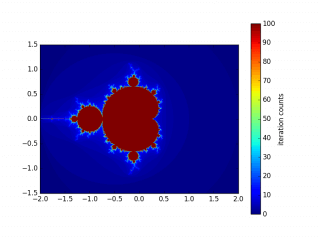1  convert *.png out.gif